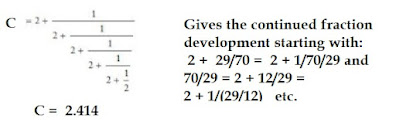## Monday, October 3, 2022

### The Algebra Of Diophantine Equations

A Diophantine equation is "an algebraic equation in one or more unknown with integer coefficients for which integer solutions are sought".  This according to the text 'What Is Mathematics?' (p. 50)  Further such an equation may have: " no solutions, a finite number of solutions, or an infinite number."   The basic template for this type of equation is:  ax + by = c.

Here, a, b and c denote integers and the equation needs to be solved for x and y, and ideally these solutions are integers, not fractions.  At this point, we have to cut to the Euclidean algorithm and how it's used.  A general theorem may be stated thus: If a is any integer, and b is any integer greater than zero, then we can always find an integer q such that:

a = b*q + r

where r is an integer satisfying the inequality:  0 < r < b

A general task is to find the “greatest common divisor” (gcd)  of a and b, called d(a,b). If d = (a,b)then positive or negative integers k and m can always be found such that:  d = ka + mb

This proceeds by successive divisions with remainders. As an example, consider the Euclidean algorithm for finding (a,b) = (61, 24). Here gcd or greatest common divisor is 1 and we find:

61 = 2*24 + 13

24 = 1*13 + 11

13 = 1*11 + 2

11 = 5*2 + 1

2 = 2*1 + 0

From the 1st equation we have: 13 = 61 - 2*24

From the end of the second result we get:

11 = 24 – 13 = 24 - 61 - 2*24 = -61 + 3*24

From the third:

2 = 13 – 11 = (61 – 2*24) – (-61 + 3*24) = 2*61 – 5*24

From the fourth:

1 = 11 – 5*2 = (-61 + 3*24) – 5(2*61 – 5*24) = -11.61 + 28*2

Apart from these operations, we can also use the Euclidean algorithm to compute continued fractions.  F
or example,  consider 169/70 and seek the continued fraction development:

169/70 = 2 + 29/70 = 2 + 1/70/29

70/29 = 2 + 12/29 = 2 + 1/ (29/12)

29/12 = 2 + 5/18 = 2 + 1/ (12/5)

12/5 = 2 + 2/5 = 2 + 1/(5/2)

And: 5/2 = 2 ½ = 2 + ½

Thus, as shown in the diagram below,  169/70 is developed with the result = 2.414Let's now see how the preceding operations can be used to solve a Diophantine equation. In particular, we want to solve: 7x + 11y = 13   We proceed by working with 7 and 11, respectively, using the Euclidean algorithm.

Thus:   11 = 1*7 + 4  and 7 = 1*4 + 3

We see remainder 4 for the first, so use the algorithm again, so: 4 = 1*3 + 1

Then we can write: (7, 11) = 1

Meanwhile, the remainder of 1 (after working on remainder 4) is dealt with, so:

1 = 4 - 3 = 4 - (7 - 4) = 2*4 - 7 = 2 (11 - 7) - 7 =  2*11 - 3*7

Therefore:   7*(-3) + 11(2) = 1   and   7*(-39) + 11(26) = 13

From the last equation we see that x = -39 and y = 26.

All other solutions can be expressed in terms of x and y -values with a remainder r (integer), so that:   x = -39 + 11r  and y = 26 - 7r

For example, let r = 1, then x = (-39) + 11 = - 28 is also a solution.  Similarly, let r = 1 and y = 26 -7
(1) = 26 - 7 = 19 is also a solution.

In summary then, the linear Diophantine equation of form ax + by = c has a solution in integers if and only if c is a multiple of (a, b). In this case, a particular solution may be found using the Euclidean algorithm.

Suggested Problems:

1)     Solve the Diophantine equation: 3x - 4y = 29

(Give at least one extra solution to the one immediately proposed)

2)Solve the Diophantine equation: 11x + 12 y = 58

(Give at least one extra solution to the one immediately proposed)